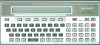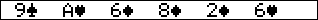##### Sharp PC-1500 : PILES Jr game

"Piles Jr" for Sharp PC-1500 or TRS-80 PC-2.
© Kemsoft 2002 (Windows version available at www.kemsoft.com).
Author : Russ Kemsley.
Unpublished.
BASIC program - 1093 bytes.RUN

Game: Piles Jr. is an addictive 6-card game of accordian. To win you must get down to one card.

Rules: You may cover up any card of the same suit or value, but the card must be either immediately to the left or the 3rd card to the left.

Play: First select the card you want to move. The computer will beep (assuming you have beep on). Then select the card you wish to cover with the first card. The computer will beep again. It it's a valid move, the cards will be rearranged accordingly. If it is not, you will get a message indicating so. Feel free to contact the author at russ@kemsoft.com.

Keys:
F1-F6 - select cards
CL - quit current game

BASIC program.

1 DATA "185C7F5C18","1C3E7C3E1C","0C4F734F0C","183C7E3C18"
10 " "CLEAR :RESTORE :DIM C(7),S(7),G\$(4):H=5:RANDOM
15 FOR I=1TO 4:READ G\$(I):NEXT I
17 WAIT 0:CLS :CURSOR 9:PRINT "Piles Jr."
20 FOR I=0TO 5
25 "RAND"WAIT 0:C=RND 13:S=RND 4
30 FOR J=0TO 5
40 IF C=C(J)AND S=S(J)THEN GOTO "RAND"
50 NEXT J
60 C(I)=C:S(I)=S:NEXT I
70 "HERE"GOSUB "DISP":GOSUB "SEL":GOTO 100
75 "DISP"CLS :WAIT 0:FOR I=0TO H
76 T\$=STR\$ (C(I)):IF C(I)=11LET T\$="J"
77 IF C(I)=12LET T\$="Q"
78 IF C(I)=13LET T\$="K"
79 IF C(I)=1LET T\$="A"
80 CURSOR (I*4+1):PRINT T\$;:GPRINT G\$(S(I));:NEXT I:RETURN
85 GOSUB "SEL":GOTO 100
90 "SEL"A\$=INKEY\$ :IF (ASC (A\$)<17OR ASC (A\$)>24)GOTO 90
95 RETURN
100 S1=(ASC (A\$)-17):BEEP 1,16,20
101 IF S1=7GOSUB "QUIT"
110 GOSUB "SEL":S2=(ASC (A\$)-17):BEEP 1,16,20
111 IF S2=7GOSUB "QUIT"
120 WAIT :IF (C(S1)<>C(S2))AND (S(S1)<>S(S2))CLS :PAUSE "That is not a legal move":GOTO "HERE"
130 WAIT :IF (S1-S2)<>3AND (S1-S2)<>1CLS :PAUSE "That is not a legal move":GOTO "HERE"
200 C(S2)=C(S1):S(S2)=S(S1)
210 C(S1)=0:S(S1)=0
220 FOR I=S1TO H-1
230 C(I)=C(I+1):S(I)=S(I+1)
240 NEXT I
500 H=H-1:IF H=0BEEP 5:CLS :CURSOR 8:PAUSE "You won!!!"
550 GOTO "HERE"
1000 "QUIT"CLS :PRINT "Quit game? (Y) (N)":GOSUB "SEL"
1010 IF ASC (A\$)=21GOTO "SURE"
1020 IF ASC (A\$)=22RETURN
1050 "SURE"CLS :PRINT "Play again? (Y) (N)"
1060 GOSUB "SEL"
1070 IF ASC (A\$)=21GOTO " "
1080 IF ASC (A\$)=22END
1090 RETURN